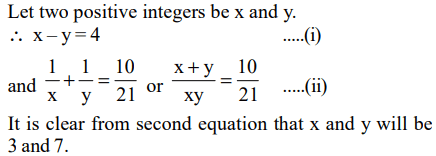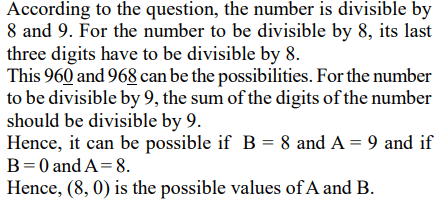## Questions on Number System for CAT Exam

1.What is the smallest number which when increased by 5 is completely divisible by 8, 11 and 24?
a) 264
b) 259
c) 269
d) None of these

Explanation: Required no. = LCM of ( 8, 11, 24 ) –5 = 264 – 5 = 259

2. Which is the least number that must be subtracted from 1856, so that the remainder when divided by 7, 12 and 16 will leave the same remainder 4
a) 137
b) 1367
c) 140
d) 172

Explanation: Suppose least no. be x
1856 - x = n (LCM of 7,12,16) + 4
or 1856 – x = n (336)+ 4
we should take n = 5 so that n(336) is nearest to 1856
and n (336) < 1856
1856 – x = 1680 + 4 = 1684
x = 1856 – 1684 = 172

3. Two positive integers differ by 4 and sum of their reciprocals is 10/21. Then one of the number is
a) 3
b) 1
c) 5
d) 21

Explanation:4. Three bells chime at an interval of 18, 24 and 32 minutes respectively. At a certain time they begin to chime together. What length of time will elapse before they chime together again?
a) 2 hours 24 minutes
b) 4 hours 8 minutes
c) 1 hours 36 minutes
d) 5 hours

Explanation: L.C.M of 18, 24 and 32 = 288
Hence they would chime together after every 288 min.
or 4 hrs. 8 min

5. For the product n(n+1)(2n+1), n $\epsilon$ N, which one of the following is not necessarily true?
a) It is always even
b) Divisible by 3.
c) Always divisible by the sum of the square of first n natural numbers
d) Never divisible by 237.

Explanation: It is clear that for n = 237 the expression n(n + 1) (2n + 1) is divisible by 237.
Hence option (d) is not necessarily true

6. The remainder obtained when a prime number greater than 6 is divided by 6 is
a) 1 or 3
b) 1 or 5
c) 3 or 5
d) 4 or 5

Explanation: It is clearly 1 or 5
Example : 7 divided by 6 leaves remainder 1
11 divided by 6 leaves remainder 5
13 divided by 6 leaves remainder 1

7. Cost of 72 hens is Rs .....96.7..... Then, what will be the cost of hen, where two digits in place of “ ....... ” are not visible or are written in illegible hand-writing?
a) Rs. 3.23
b) Rs. 5.11
c) Rs. 5.51
d) Rs. 7.22

Explanation: Multiply each option by 72 and find out the result which matches the visible digits.
Clearly we see 72 * 5.51 = 396.72

8. Three consecutive positive even numbers are such that thrice the first number exceeds double the third number by 2 then the third number is
a) 10
b) 14
c) 16
d) 12

Explanation: Let x - 2, x, x + 2 be the 3 consecutive numbers
then, 3 (x – 2) = 2 (x + 2) + 2 (according to the question)
or 3x - 6 = 2x + 6
or x = 12
Hence, the 3rd no. is 14

9. $5^{6}-1$ is divisible by
a) 13
b) 31
c) 3
d) None of these